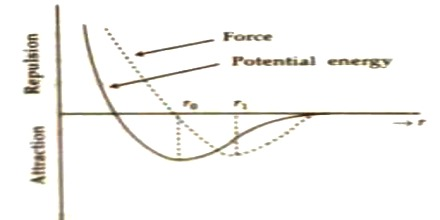# Nature of Intermolecular Force

How the intermolecular force and potential energy between two molecules are changed due to the change of distance between them are discussed below.

Suppose, interatomic force and interatomic distance between two molecules are respectively F and r. There is a strong relation between these quantities. In figure intermolecular force versus distance and potential energy versus distance have been shown by graphs.When the distance between molecules becomes large, then there exists very small interatomic force (as in case of gas molecules). As the molecules gets closer and closer i.e., distance between than starts decreasing, attractive force between them starts increasing and reaches to a maximum value. After that if, the distance decreases further, then attractive force also starts decreasing. That means, then interatomoic repulsive force becomes active. When value of r tends to r0, then force becomes zero.

In this situation, interatomic attractive and repulsive forces become equal. It is evident from potential energy versus distance curve that potential energy decreases as the distance between atoms/molecule decreases and at r = r0, the Potential energy becomes minimum. It is the natural law that a system becomes stable, when its potential energy becomes minimum. So, r = r0 position is called the equilibrium position and distance r0 is called equilibrium or stable distance molecules of different materials have different r0 values.